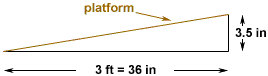Quandaries and Queries This is a question about incline percentage. On an exercise machine if the elevation is raised 3 and one half inches from a level position then what would be the incline percentage? Thank You Hi, I expect that the incline is measured as the elevation divided by the length of the platform. The incline percentage is then this quantity, expressed as a percentage. For example, suppose that the platform is 3 feet long as in the diagram below.In this case the elevation at the high end is 3.5 inches and the length of the platform is 36 inches so the incline (sometimes called slope of grade) is 3.5/36 = 0.0972 or, as a percentage, 9.72%. Penny Go to Math Central